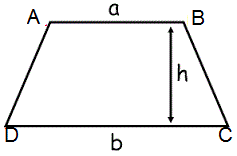# Trapezium Calculator

Calculate the various properties of Trapezium for given values.

Area of Trapezium/Trapezoid:
[ ½×(sum of parallel sides)×(distance between them) ]
 Enter the size of parallel side 1 : Enter the size of parallel side 2 : Enter the distance between parallel sides :
 Area of Trapezium =

Perimeter of Trapezium/Trapezoid:
[ (sum of all four sides) ]
 Enter the length of the sides :
 Perimeter of Trapezium =

A Trapezium is a quadrilateral with one pair of parallel sides.

Both UK and US definitions of trapezoid and trapezium are swapped over.

The trapezium is called a trapezoid in the UK.### Formulas

Area :[ ½×(sum of parallel sides)×(distance between them) ] Perimeter:[ (sum of all four sides) ]